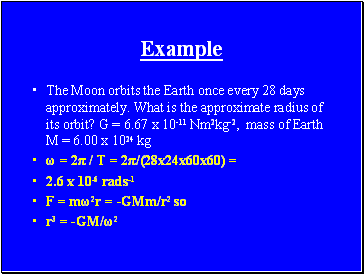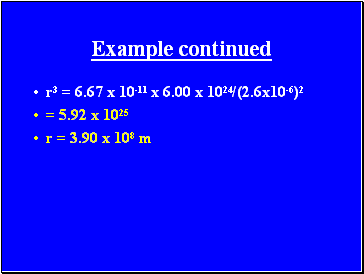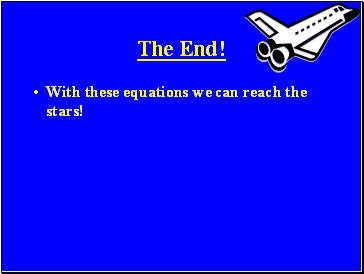# Gravity and Circular Motion RevisionPage 3

#### WATCH ALL SLIDES

T = 2π / ω

and should be calculated in

seconds

Slide 24## Example

The Moon orbits the Earth once every 28 days approximately. What is the approximate radius of its orbit? G = 6.67 x 10-11 Nm2kg-2, mass of Earth M = 6.00 x 1024 kg

ω = 2π / T = 2π/(28x24x60x60) =

F = mω2r = -GMm/r2 so

r3 = -GM/ω2

Slide 25Example continued

r3 = 6.67 x 10-11 x 6.00 x 1024/(2.6x10-6)2

= 5.92 x 1025

r = 3.90 x 108 m

Slide 26The End!

With these equations we can reach the stars!

Go to page:
1  2  3